# Lens and Mirror Calculations and Formulae

The formula for lens makers is as follows: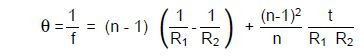Where:
f = Lens focal length
n = Index of refraction of lens material
R1 = Radius of curvature of 1st surface
R2 = Radius of curvature of 2nd surface
t = Lens thickness

Figure 1 shows a curvature for which R2 is negative.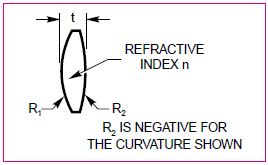Figure 1. Shows a curvature for which R2 is negative

## Focal Length of Spherical Mirror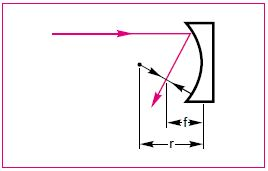Figure 2. The focal length of a spherical mirror

The formula for determining the focal length of a spherical mirror (Figure 2) is: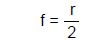Where r = Radius of curvature of mirror

## Effective Focal Length of Two Thin Lenses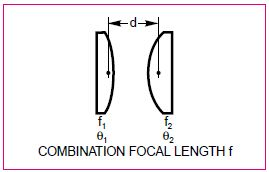Figure 4. Effective focal length of two thin lenses

Effective focal length (Figure 3) of two thin lenses can be determined using the following formula: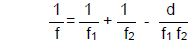θ= θ1 + θ2 - θ1 θ2 d

Where:
f1 = Focal length of lens 1
f2 = Focal length of lens 2
θ1 = Power of lens 1
θ2 = Power of lens 2
d = Distance between lenses

## Image Location and Magnification Formulae, and Graphical Ray Tracing

The Gaussian Lens Formula is as follows: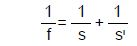Magnification is equal to SI/S

Where:
f = Lens focal length
S = Object distance
SI= Image distance

The Newtonian Lens Formula is as follows:

XXI=f2

Magnification is =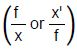The lens formulae and graphical techniques can be used with input and output on the same side of the optic. Ray tracing is shown graphically in Figure 4.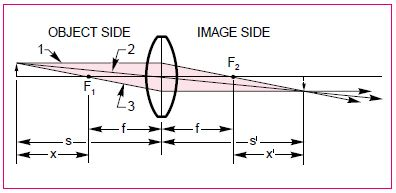Figure 4. Graphical Ray Tracing

Figure 5 shows the re-imaging of a source using a collimating condenser and refocusing lens.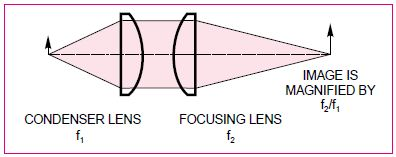Figure 5. Re-imaging a source with a collimating condenser and refocusing lens

## Focused Spot Size

Uniform illumination of a Diffraction Limited Lens is expressed as follows: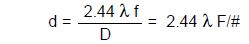Illumination with a laser beam using a Gaussian intensity profile for a Diffraction Limited Lens is expressed as follows: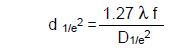Oriel Instruments, a Newport Corporation brand, was founded in 1969 and quickly gained a reputation as an innovative supplier of products for the making and measuring of light. Today, the Oriel brand represents leading instruments, such as light sources covering a broad range, from UV to IR, pulsed or continuous, and low to high power.

Oriel also offers monochromators and spectrographs, as well as flexible FT-IR spectrometers, which make it easy for users across many industries to build instruments for specific applications. Oriel is also a leader in the area of Photovoltaics with its offering of solar simulators, that allow you to simulate hours of solar radiation in minutes. Oriel continues to bring innovative products and solutions to Newport customers around the world.This information has been sourced, reviewed and adapted from materials provided by Oriel Instruments.

## Citations

• APA

Oriel Instruments. (2019, July 24). Lens and Mirror Calculations and Formulae. AZoOptics. Retrieved on July 03, 2020 from https://www.azooptics.com/Article.aspx?ArticleID=893.

• MLA

Oriel Instruments. "Lens and Mirror Calculations and Formulae". AZoOptics. 03 July 2020. <https://www.azooptics.com/Article.aspx?ArticleID=893>.

• Chicago

Oriel Instruments. "Lens and Mirror Calculations and Formulae". AZoOptics. https://www.azooptics.com/Article.aspx?ArticleID=893. (accessed July 03, 2020).

• Harvard

Oriel Instruments. 2019. Lens and Mirror Calculations and Formulae. AZoOptics, viewed 03 July 2020, https://www.azooptics.com/Article.aspx?ArticleID=893.